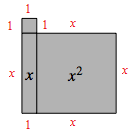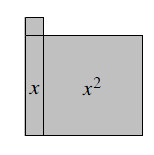### Home > MC2 > Chapter 3 > Lesson 3.1.4 > Problem3-38

3-38.

Sketch the algebra tile shape below on your paper. Write an expression for the perimeter of the shape, then find the perimeter for each of the given values of $x$.

Find the perimeter as you did in problems 3-29 and 3-20, including $x$ as part of the expression.

$P = 4x + 4$1. $x = 7$ units

Substitute $7$ for $x$ in the expression of the perimeter.

Evaluate the expression.
$4(7) + 4 = ?$

$32$ units.

1. $x = 5.5$ units

Substitute $5.5$ for $x$ in the expression of the perimeter.

Evaluate the expression.

$26$ units.

1. $x = \frac { 7 } { 3 }$ units

See parts (a) or (b).